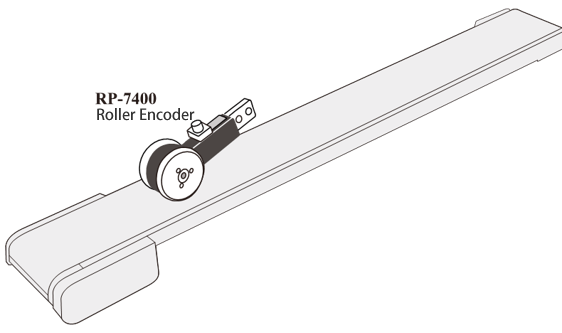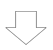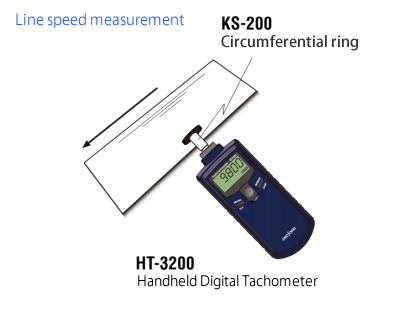Our Roller encoder RP-7400 enables line speed and distance measurement by contacting the roller with the measured object. We explain why output pulses such as 120 and 1200 P/R is necessary for speed measurement in terms of measurement mechanism.

Since the speed is the distance traveled per unit time, it is calculated as Speed = Travelling distance / Time taken.When measuring Line speed by Roller encoder RP-7400,
Travelling distance = Rotation of roller
Speed = Distance per 1 pulse x Number of output pulses/ Time takenSpeed per second (mm)?
Number of output pulses/ Time taken is equivalent to Frequency (output pulses per second).
Thus, Speed (mm/s) = Distance per 1 pulse x Number of output pulses/ Time taken is equivalent to (Circumferential length of roller (mm)/ Number of pulse per rotation) x Frequency.
If Circumferential length (mm)/ Number of pulses is “1”, Output frequency of Encoder equals to Circumferential speed.
Since Circumferential length of RP-7400 is 200 mm, circumferential speed (mm/s) can be represented by frequency counter if selecting 200 P/R.
If number of pulses per second is measured by pulse counter, number of counts will be represented as frequency. Thus, if pulse counter has 1 second gate function, circumferential speed can be obtained from frequency.
* Since the unit of measurement resolution is 1 Hz, the unit of speed resolution is 1 mm/s.

Speed per minute (m)?
It is also calculated by Speed (m/min) = Distance per 1 pulse x Number of output pulses/ Time taken, however, the unit of length and time is different from the above mentioned.
The first half of equation is Distance per 1 pulse = (200/ Number of pulses per rotation) / 1000, the latter half of equation is Number of output pulses/ Time = Number of pulses per minute = Frequency x 60.
Then, Speed (m/min) = (200/ Number of pulses per rotation) / 1000) x Frequency x 60.
Further, if numerical values are lumped together,
Speed (m/min) = (200 x 60/ 1000/ Number of pulses per rotation) x Frequency
= (12/ Number of pulses per rotation) x Frequency
If the number of pulses per rotation of the roller is 1, 10, 100, etc. times of 12, the speed can be expressed as 1, 1/10, 1/100 times of frequency.

Using RP-7400 (120 P/R),
Speed (m/min) = Frequency x 0.1
Using RP-7400 (1200 P/R),
Speed (m/min) = Output frequency x 0.01
And using frequency counter or pulse counter with 1 second gate, Circumferential speed (m/min) can be measured.
Since the unit of measurement resolution of pulse counter with 1 second gate is 1 Hz, the speed resolution is as follow:

• 0.1 m/min (120 P/R)
• 0.01 m/min (1200 P/R)

Please select the appropriate pulse of Roller Encoder such as 120/1200 or 200 according to the unit of speed.

Supplement
On circumferential speed measurement, circumferential ring size for HT-3200 and HT-5500 is fixed based on the above calculation method.
The basic calculation is Speed (m/min) = Distance per 1 pulse x Number of output pulses/ Time taken.

As for HT, number of pulse per rotation is fixed as 1,
Distance per pulse is Circumferential length of circumferential ring.
Number of output pulses/ Time taken is Rotation speed (r/min).
If circumferential length of circumferential ring is 1m, rotation speed value equals to circumferential speed.
As for HT-3200, circumferential speed can be taken by using circumferential ring KS-200 (circumferential length 100 mm) and changing value to 1/10.
If you would like to measure mm/s,
Number of output pulses/ Time taken is equal to Rotation speed (r/min) ÷ 60.
Circumferential speed (mm/s) can be taken by using circumferential ring KS-100 (circumferential length 60 mm).(HO)